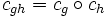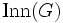# Question:Inner automorphism automorphism

(diff) ← Older revision | Latest revision (diff) | Newer revision → (diff)
A: This basically is a straightforward verification that involves some symbol manipulation. See group acts as automorphisms by conjugation, which not only shows that conjugations by elements are automorphisms, but also that the conjugation by$gh$ is the composite of conjugations by$g$ and$h$. In other words, if$c_g$ denotes conjugation by$g$, we have$c_{gh} = c_g \circ c_h$ for all$g,h \in G$.
We thus obtain a homomorphism of groups from$G$ to$\operatorname{Aut}(G)$ (the automorphism group of$G$) sending each$g \in G$ to the conjugation map$c_g$. The kernel of this is the center$Z(G)$ and the image is the inner automorphism group$\operatorname{Inn}(G)$. We thus have, from the first isomorphism theorem, that$\operatorname{Inn}(G) \cong G/Z(G)$.